Home
Hostname: page-component-99c86f546-5rzhg Total loading time: 0.168 Render date: 2021-12-07T15:54:05.666Z Has data issue: true Feature Flags: { "shouldUseShareProductTool": true, "shouldUseHypothesis": true, "isUnsiloEnabled": true, "metricsAbstractViews": false, "figures": true, "newCiteModal": false, "newCitedByModal": true, "newEcommerce": true, "newUsageEvents": true }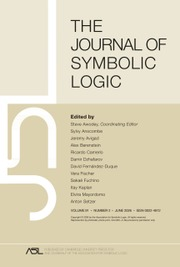The Journal of Symbolic Logic

# FACTORIALS OF INFINITE CARDINALS IN ZF PART II: CONSISTENCY RESULTS

Published online by Cambridge University Press:  04 November 2019

## Abstract

For a set x, let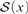${\cal S}\left( x \right)$ be the set of all permutations of x. We prove by the method of permutation models that the following statements are consistent with ZF:

(1) There is an infinite set x such that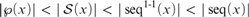$|\wp \left( x \right)| < |{\cal S}\left( x \right)| < |se{q^{1 - 1}}\left( x \right)| < |seq\left( x \right)|$, where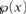$\wp \left( x \right)$ is the power set of x, seq (x) is the set of all finite sequences of elements of x, and seq1-1 (x) is the set of all finite sequences of elements of x without repetition.

(2) There is a Dedekind infinite set x such that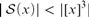$|{\cal S}\left( x \right)| < |{[x]^3}|$ and such that there exists a surjection from x onto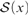${\cal S}\left( x \right)$.

(3) There is an infinite set x such that there is a finite-to-one function from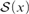${\cal S}\left( x \right)$ into x.

## Keywords

Type
Articles
Information
The Journal of Symbolic Logic , March 2020 , pp. 244 - 270

## Access options

Get access to the full version of this content by using one of the access options below. (Log in options will check for institutional or personal access. Content may require purchase if you do not have access.)

## References

Birkhoff, G., Lattice Theory, third ed., American Mathematical Society Colloquium Publications, vol. 25, American Mathematical Society, Providence, R. I., 1967.Google Scholar
Dawson, J. W. Jr. and Howard, P. E., Factorials of infinite cardinals. Fundamenta Mathematicae, vol. 93 (1976), pp. 185195.Google Scholar
Forster, T., Finite-to-one maps, this Journal, vol. 68 (2003), pp. 12511253.Google Scholar
Grätzer, G., General Lattice Theory, second ed., Birkhäuser, Basel, 1998.Google Scholar
Halbeisen, L., Combinatorial Set Theory: With a Gentle Introduction to Forcing, second ed., Springer Monographs in Mathematics, Springer, Cham, 2017.CrossRefGoogle Scholar
Halbeisen, L., A weird relation between two cardinals. Archive for Mathematical Logic, vol. 57 (2018), pp. 593599.CrossRefGoogle Scholar
Halbeisen, L. and Shelah, S., Consequences of arithmetic for set theory, this Journal, vol. 59 (1994), pp. 3040.Google Scholar
Halbeisen, L. and Shelah, S., Relations between some cardinals in the absence of the axiom of choice. Bulletin of Symbolic Logic, vol. 7 (2001), pp. 237261.CrossRefGoogle Scholar
Howard, P. and Rubin, J. E., Consequences of the Axiom of Choice, Mathematical Surveys and Monographs, vol. 59, American Mathematical Society, Providence, R. I., 1998.Google Scholar
Jech, T., The Axiom of Choice, Studies in Logic and the Foundations of Mathematics, vol. 75, North-Holland, Amsterdam, 1973.Google Scholar
Rubin, J. E., Non-constructive properties of cardinal numbers. Israel Journal of Mathematics, vol. 10 (1971), pp. 504525.CrossRefGoogle Scholar
Shen, G., Generalizations of Cantor’s theorem in ZF. Mathematical Logic Quarterly, vol. 63 (2017), pp. 428436.Google Scholar
Shen, G., A note on strongly almost disjoint families. Notre Dame Journal of Formal Logic, accepted, 2019.Google Scholar
Shen, G. and Yuan, J., Factorials of infinite cardinals in ZF, Part I: ZF results, submitted, 2019.Google Scholar
Sonpanow, N. and Vejjajiva, P., Factorials and the finite sequences of sets. Mathematical Logic Quarterly, vol. 65 (2019), pp. 116120.CrossRefGoogle Scholar
Specker, E., Verallgemeinerte Kontinuumshypothese und Auswahlaxiom. Archiv der Mathematik, vol. 5 (1954), pp. 332337.Google Scholar
Tachtsis, E., On the existence of permutations of infinite sets without fixed points in set theory without choice. Acta Mathematica Hungarica, vol. 157 (2019), pp. 281300.CrossRefGoogle Scholar
Truss, J., Dualisation of a result of Specker’s. Journal of the London Mathematical Society, vol. 6 (1973), pp. 286288.CrossRefGoogle Scholar
Vejjajiva, P. and Panasawatwong, S., A note on weakly Dedekind finite sets. Notre Dame Journal of Formal Logic, vol. 55 (2014), pp. 413417.CrossRefGoogle Scholar
2
Cited by

# Send article to Kindle

Note you can select to send to either the @free.kindle.com or @kindle.com variations. ‘@free.kindle.com’ emails are free but can only be sent to your device when it is connected to wi-fi. ‘@kindle.com’ emails can be delivered even when you are not connected to wi-fi, but note that service fees apply.

Find out more about the Kindle Personal Document Service.

FACTORIALS OF INFINITE CARDINALS IN ZF PART II: CONSISTENCY RESULTS
Available formats
×

# Send article to Dropbox

To send this article to your Dropbox account, please select one or more formats and confirm that you agree to abide by our usage policies. If this is the first time you use this feature, you will be asked to authorise Cambridge Core to connect with your <service> account. Find out more about sending content to Dropbox.

FACTORIALS OF INFINITE CARDINALS IN ZF PART II: CONSISTENCY RESULTS
Available formats
×

# Send article to Google Drive

To send this article to your Google Drive account, please select one or more formats and confirm that you agree to abide by our usage policies. If this is the first time you use this feature, you will be asked to authorise Cambridge Core to connect with your <service> account. Find out more about sending content to Google Drive.

FACTORIALS OF INFINITE CARDINALS IN ZF PART II: CONSISTENCY RESULTS
Available formats
×
×